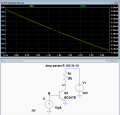# Does BJT's collector resistor value changes the value of Ic?

Thread Starter

#### Smilehoho

Joined Feb 21, 2017
2
Hello. I am having a hard time to understanding the Ic value through NPN transistor.
If Beta of NPN transistor is 100, Does Ic determined to 100*Ib regardless from Rc (Resistor from collector) or Rc does affect Ic?

I uploaded a part of graph from my textbook.
At the graph of right below. What I concluded is ' Only if Rc is 0, than the intersection of load line and Characteristic graph of Collector-emitter is become Quotient point'

Let's say I change the value of Rc to something higher than Rc (which is +Rc). Than the intersection point moves from Q to B. which is clearly not a 100*Ib ( Beta * Ib ).

what my textbook says is after you calculate Ic from [beta*Ib]. that is the Ic regardless Rc. if so, if Rc value limit the current below the Ic. what will happen?

thank you for reading my question.

#### crutschow

Joined Mar 14, 2008
26,742
The transistor collector equivalent resistance is high but not infinite, so the current does change with a change in collector load resistance (as long as the transistor doesn't saturate) but only slightly.

Here's an LTspice simulation of that:
The collector current only varies about 0.4 mA as the collector resistance, R1, goes from 100Ω to 3kΩ, causing the collector voltage to go from about 19.6V to 10.3V.
The equivalent transistor collector resistance is thus about 22kΩ......
if Rc value limit the current below the Ic. what will happen?
The transistor goes into saturation.

Thread Starter

#### Smilehoho

Joined Feb 21, 2017
2
The transistor collector equivalent resistance is high but not infinite, so the current does change with a change in collector load resistance (as long as the transistor doesn't saturate) but only slightly.
So in summary, it does affect the value of Currents of collector, but it is small enough to be negligible.
Thank you for the answer.

#### MrAl

Joined Jun 17, 2014
8,063
Hi,

The simplified version is that it does not change the collector current, but ONLY when the transistor is in the ACTIVE region (sometimes referred to as the linear region).
There are two extremes when this does not work at all, and that is when the transistor goes into saturation and when the transistor goes into cutoff.

Keep in mind this is the simplified version which is used in some school studies just to study the BASIC operation of a transistor. The collector current is then said to be due to the base current times the Beta.
So when they say that iC=iB*Beta, they are not really wrong, they are using the simplified view which is not always as accurate as a full analysis.

A full analysis of a transistor circuit even in static operation is more complicated then they like to get into sometimes so they use approximate models to show the basic operation, then later in the course may show more complicated models which take more things into account like saturation.